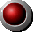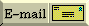Computer Power
 Estimating the power used by all US computers The population of the US is about 260 million people.  Many have computers and many do not.  Let us estimate that there are 50 million computers in the US. A computer and its monitor each consume about 100 watts.  Let us estimate that each US computer system is turned on 50% of the time.  Therefore, one-half of the 200 turned-on watts is the around-the-clock average power consumption, namely 100 watts. Then the average electrical power used for running the 50 million computers is 5 billion watts, the full power output of five very large power stations. By comparison, during the period 1985 to 1997, the US electrical power demand grew about 90 billion watts.
To main Energy Advocate IndexYour comments & questions, please